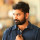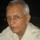Coffee Room
Discuss anything here - everything that you wish to discuss with fellow engineers.
12889 Members
Join this group to post and comment.# A steel ball of 10 mm diameter at 1000 K is required to be cooled to 350 K by immersing it in a water environment at 300 K

A steel ball of 10 mm diameter at 1000 K is required to be cooled to 350 K by immersing it in a water environment at 300 K. The convective heat transfer coefficient is 1000 W m/ 2 -K. Thermal conductivity of steel is 40 W/m-K. The time constant for the cooling process τ is 16 s. The time required (in seconds) to reach the final temperature is ______

Can anyone please post the correct answer? Also steps to arrive at the correct answer?Since this is a dynamic situation it is not possible for the whole steel ball to be at one temperature as the heat has to flow from the centre of the ball to the surface, which has a time factor due to the conductivity of steel.

The area for convection is the surface area a of the sphere. the mass m of he ball is easily calculated from the size of the ball. Speciific heat of steel Ccan be got from tables. The Temperature difference is (1000-350). The total heat removed is 650mCp.

The mean temperature difference is ((1000-350)+(350-300))/2

From the area a and the mean temp diff and the rate of convective transfer, the time can be got.

However this does not include the perturbation caused by the time for conductive transfer of heat from the centre of the ball to the periphery.

You have to set up the appropriate differential equations to take into account this.Is this an academic problem needing theoretically correct analytic solution or a practical problem? If a good approximation is sufficient one can ignore the calculus part. Fora small object like the 10 mm dia metal ball one can safely ignore the conductive transfer within the ball. Then it becomes a trivial arithmetic problem.

Share this content on your social channels -